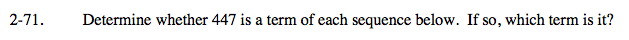Home > A2C > Chapter 2 > Lesson 2.1.5 > Problem2-71

2-71.
1. Determine whether 447 is a term of each sequence below. If so, which term is it? Homework Help ✎

1. t(n) = 5n − 3

2. t(n) = 24 − 5n

3. t(n) = −6 + 3(n − 1)

4. t(n) = 14 − 3n

5. t(n) = −8 − 7(n − 1)447 = 5n − 3

90 = n

Yes, 447 is the 90th term.No, 447 is not a term of t(n).447 = −6 + 3n − 3No, 447 is not a term of t(n).